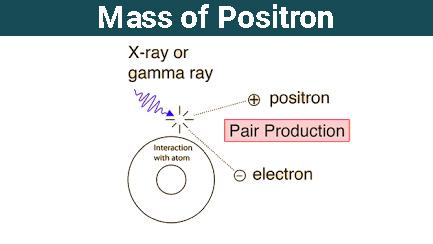# Positron - Mass of Positron

## What is a Positron?

Positron is a subatomic particle whose mass is the same as that of electron and numerically equal but positively charged particle.

The collision of low-energy positron and a low-energy electron results in the formation of two or more gamma rays photon and this process is known as electron-positron annihilation. The two main processes of production of positron are radioactive decay and the pair production. The interaction between energetic photons with an atom in a material is known as pair production.

The positron was discovered by Carl D. Anderson in the year 1932. It is represented by e+, β+. It is an antiparticle called antielectron. Its mass is the same as the mass of an electron.Mass of Positron

Electrons, Protons, and Neutrons are known as the fundamental particles of atoms. Electrons are placed in certain orbits surrounding the nucleus. Protons and neutrons are placed within the nucleus that lies at the centre of the atom. But, that does not mean that only these three particles are present in an atom. There is another particle which is involved in the transition of one particle to another with some charge.

## What is Mass of Electron?

The mass of an electron is 9.10938356 × 10-31 kilograms

Mass of neutron is 1.0086654 a.m.u. or 1.6749 x 10-27 kilograms.

The mass of electron is negligible compared to the mass of a proton. For example, the mass of the helium nucleus is about 4 times the mass of proton but the charge on it is only +2e. The proton mass is + 1. The proton has about 1 amu mass. This amounts to approximately 1.66 x 10-24 g in grams, about 1837 times the electron’s mass. A proton’s relative mass is 1. A neutron’s relative mass is 1. An electron’s relative weight is 1/1840.

## Recommended Videos## Mass of Positron in Amu

• As we know, the mass of a proton is 1.007593 amu.
• Similarly, mass of an electron is 0.000548756 amu or 9.10093897 x 10-31 kg.
• The mass of positron is equal to the mass of electron i.e., 0.000548756 amu.
• The exact value of the mass of the positron is about 0.511 MeV/c2 or 9.11 x 10-31 kg.

Since positron is produced in radioactive decay by departing neutron, their kinetic energy is supported by their motion. According to the concept of conservation of energy and momentum, the positron loses some of its kinetic energy when it collides with the other particles.  They come to rest position as a result of energy loss. The positron particles are ready to participate in an annihilation reaction after losing kinetic energy.

## Rest Mass of Positron

The encounter of a positron with an electron obeys the momentum or laws of energy conservation. These two particles together annihilate each other and transform all their mass to energy to form two photons.

The momentum of the system is zero because the positron and electron are almost at rest before annihilation and remain same after annihilation. Therefore, two photons travel in opposite directions and the net momentum is zero. The rest mass of the electron and positron are same that is 511 keV, therefore each photon has 511 keV of energy.

1. Who discovered positron?
Ans: Carl D. Anderson discovered positron.

2. Positrons are also called ________.
Ans: Antielectron

3. Give one application of positrons.
Ans: Positrons combined with antiprotons are used to study the properties of antihydrogen.

4. What is the approximate mass of an electron?
Ans: An electron’s invariant mass is about 9.109×10−31 kilograms or 5.489×10−4 nuclear units.

5. Who discovered the mass of electron?
Ans: The experiment by Thomson and the electron’s charge-to-mass ratio. The first is Joseph John Thomson’s experiment, which first showed that atoms are actually made up of charged particle aggregates.

6. Why do electrons have mass?
Ans: Electrons have mass because they interact with the field of Higgs. If it were massless, the messenger particles or force carriers would be called as a photon or gluon.

7. Do electrons have more mass than protons?
Ans: Protons and neutrons have approximately the same mass, but they are both much more massive than electrons (approximately 2,000 times as massive as an electron).

8. Are electrons larger than protons?
Ans: It relies on the greater meaning of what you mean. A proton is more massive than an electron about 1835 times.

To know more about the positron and any other Chemistry related topic, visit BYJU’S.